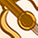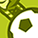Announcements

# AURIX™ Forum DiscussionsLevel 2
Level 2

Hello Everyone,
I have a problem about the fast sqrt in the 3.1 of
the docment said:Yn+1 = Yn * (1.5 – (X*2)* Yn^2), I don't know why it is like this.
I know the fast alg of sqrt with div is like that: Yn+1=0.5*(Yn+X/Yn), it is implemented with div, so, it is not fast.
furthermor, I kown the fast alg of invSqrt is:Yn+1=Yn * (1.5 – (X*0.5)* Yn^2), it avoid div, so, it is fast. and it is very similar to infineion's fast sqrt.
I really don't know how to conclude formula of infineion's fast sqrt, Is there anyone would like to tell me?or, tell me how to find the reference papers?
4 RepliesLevel 2
Level 2

# Re: About the fast sqrt

it's so pity, nobody tell me how to doLevel 6
Level 6# Re: About the fast sqrt

Hi Icework. The TriCore DSP Optimization Guide is from 2003 and has not been updated. Many of the TC2xx instructions are not reflected in it, including the integer divide unit (DIV), and the inverse square root instruction QSEED. I would see what the compiler generates before resorting to hand-coded assembly.Level 2
Level 2

# Re: About the fast sqrt

my compiler: tricore-gcc (HighTec Release HDP-v4.9.1.0-infineon-2.0-df254e8) 4.9.4 build on 2018-04-18
I just want to know how to conclude the formula, so, I think the compiler is not the key for my questionLevel 5
Level 5# Re: About the fast sqrt

Hi Shaquille,

This one had me confused for a while as well. The confusion starts with the formula (ignore the PHP Code prefix):

[PHP]
Yn1 = Yn*(1.5–(2*sX)*Yn^2)
[/PHP]

Whereas you would expect the following inverse square root formula instead:

[PHP]
Yn1 = Yn*(1.5–(sX/2)*Yn^2)
[/PHP]

So initially it appears to make no sense. Let's start with a breakdown of the formula:

[PHP]
Yn1 = Yn*(1.5–(2*sX)*Yn^2)
[/PHP]

This was unlucky steno perhaps. What is being referred to is this assembly statement:

`MOV sK,sX`

And particularly the comment behind it that says that sK is implicitly being scaled to twice the size of sX because it will be used in Q2.14 calculations. This is what the factor 2 is referring to. But even then the formula still doesn't seem to make sense. How can half sX be scaled upwards by a factor 4 while at the same time constant 1.5 is unchanged? That is mathematically impossible. Yet still, when you code this bit of assembly, it rings true every time.

I think the mistake lies in some of the comments, in this snippet of assembly:

`MULR.Q    tmp,sY u,sY u,#1SH        tmp,tmp,#-1 MSUBRS.Q  tmp,d8,tmp u,sK u,#1`

For the first line of code the comment says it will be formatted in Q1.15. This is probably not the case. It - the result of the calculation - must be Q3.13 which is then scaled to Q4.12 in the next statement. The third statement uses Q3.13 has its outcome. The multiplication itself presumes its members are in Q2.14. This was the reason why sX was implicitly scaled upwards by a factor 2. For the same reason Yn^2 must therefore be scaled down by a factor 4. So what is in fact being calculated in the third statement (Q3.13) is this:

[PHP]
0.5–(2*X)*((Yn^2)/4)
0.5–(sX/2)*Yn^2)
[/PHP]

And then it is starting to make sense. After the iterations complete, the exit-format is in Q2.14. By then the loop will have converged to 1/2z. The scaling by 1/2 is because prior to exit Q3.13 is scaled to Q2.14.

The exit formula (the last three assembly statements) is this one:

[PHP]
SQRT(sX) = ((2*sX-1)/(2*SQRT(sX))) + 1/(2*SQRT(sX))
= (2*sX - 1 + 1)/(2*SQRT(sX))
= sX/SQRT(sX)
[/PHP]

I therefore think it could also simply have been this single statement instead:

`MULR.Q		%d3,%sYu,%sKu,1`

As this calculates the following in one go:

[PHP]
SQRT(sX) = (2*sX)/(2*SQRT(sX))) = sX/SQRT(sX)
[/PHP]

Best regards,

Henk-Piet Glas

Principal Technical Specialist
Embedded SoftwareThis widget could not be displayed.